## Want to keep learning?

This content is taken from the RMIT University's online course, Online Business: Pricing for Success. Join the course to learn more.
4.7

## RMIT University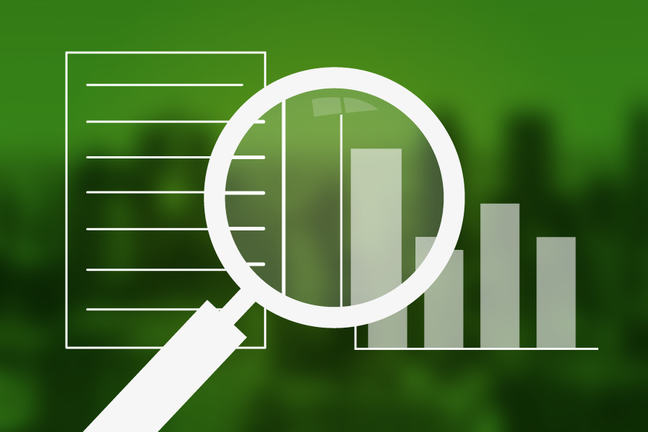Impact of selling price and profit in breakeven

# Impact of selling price and profit in breakeven

As mentioned earlier, the breakeven calculation requires you to know your fixed and variable costs, the way they respond to change in volume, and the relationship to the change in profit.

Now that you have both your fixed and variable costs per unit, it’s time to look at the relationship of the change with your profit; and ultimately identify the number of units you must sell in order to breakeven.

The first stage of a breakeven analysis is to gather three pieces of data:

• Fixed and variable costs
• Fixed and variable costs per unit
• Selling price per unit

Once you have these three pieces, you are ready to calculate your breakeven.

Calculating the Selling Price

If you are unsure of your selling price, then you will need to calculate this by using the following simple equation.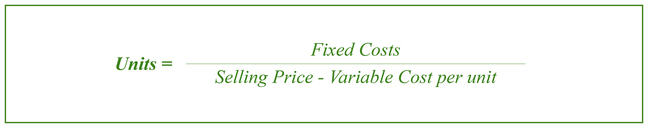Staying with the earlier example, remember there were the following:

• Fixed costs = $10,000 • Unit = 1,000 • Variable cost per unit =$2

So just input the information to establish the selling price: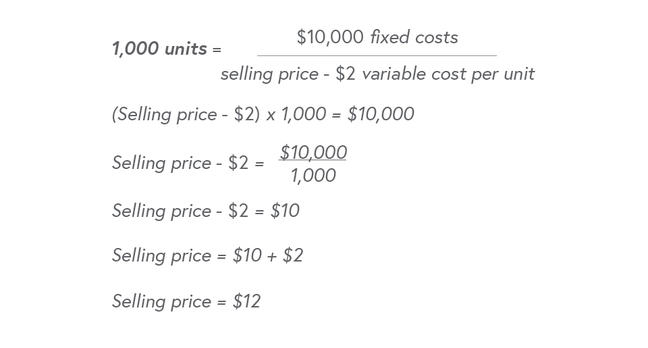Based on the calculation, the sell price would be $12.00 per item to reach breakeven. You can cross check to make sure the equation is correct by applying a formula that will calculate total sales vs. costs: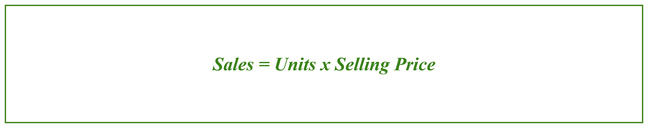Sales = 1,000 x$12

Sales = $12,000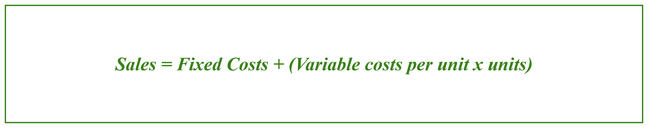Calculating your Profit Having identified the selling price, you can use the breakeven to determine the level of sales required to achieve the desired profit. The business owner wanted$20,000 profit from product sales. You can apply the following breakeven formula to determine the number of units.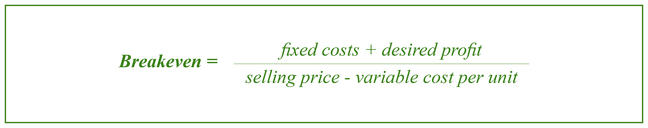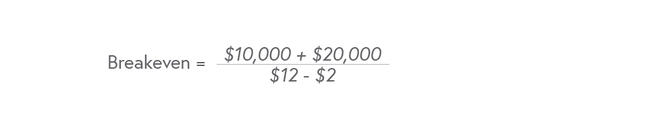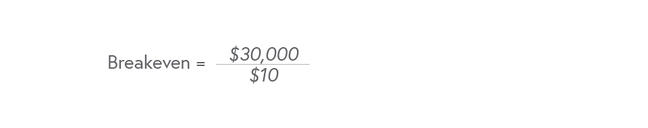Breakeven = 3,000 units to break-even AND achieve a desired profit of \$20,000.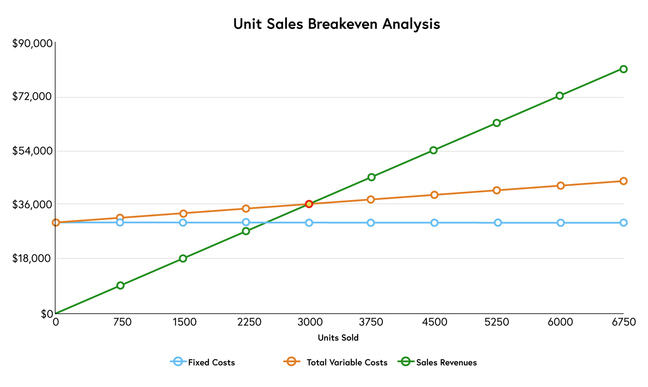Activity

What are some of the advantages and/or disadvantages when conducting a breakeven analysis?